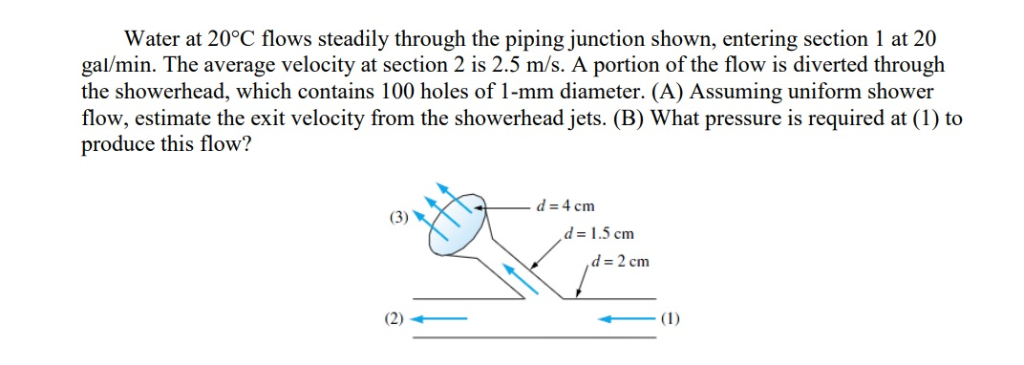# Question Solved1 AnswerWater at 20°C flows steadily through the piping junction shown, entering section 1 at 20 gal/min. The average velocity at section 2 is 2.5 m/s. A portion of the flow is diverted through the showerhead, which contains 100 holes of 1-mm diameter. (A) Assuming uniform shower flow, estimate the exit velocity from the showerhead jets. (B) What pressure is required at (1) to produce this flow? Water at 20°C flows steadily through the piping junction shown, entering section 1 at 20 gal/min. The average velocity at section 2 is 2.5 m/s. A portion of the flow is diverted through the showerhead, which contains 100 holes of 1-mm diameter. (A) Assuming uniform shower flow, estimate the exit velocity from the showerhead jets. (B) What pressure is required at (1) to produce this flow? d-4 cm d1.5 cm d 2 cmL8UQTF The Asker · Mechanical Engineering

Water at 20°C flows steadily through the piping junction shown, entering section 1 at 20 gal/min. The average velocity at section 2 is 2.5 m/s. A portion of the flow is diverted through the showerhead, which contains 100 holes of 1-mm diameter. (A) Assuming uniform shower flow, estimate the exit velocity from the showerhead jets. (B) What pressure is required at (1) to produce this flow?Transcribed Image Text: Water at 20°C flows steadily through the piping junction shown, entering section 1 at 20 gal/min. The average velocity at section 2 is 2.5 m/s. A portion of the flow is diverted through the showerhead, which contains 100 holes of 1-mm diameter. (A) Assuming uniform shower flow, estimate the exit velocity from the showerhead jets. (B) What pressure is required at (1) to produce this flow? d-4 cm d1.5 cm d 2 cm
More
Transcribed Image Text: Water at 20°C flows steadily through the piping junction shown, entering section 1 at 20 gal/min. The average velocity at section 2 is 2.5 m/s. A portion of the flow is diverted through the showerhead, which contains 100 holes of 1-mm diameter. (A) Assuming uniform shower flow, estimate the exit velocity from the showerhead jets. (B) What pressure is required at (1) to produce this flow? d-4 cm d1.5 cm d 2 cm